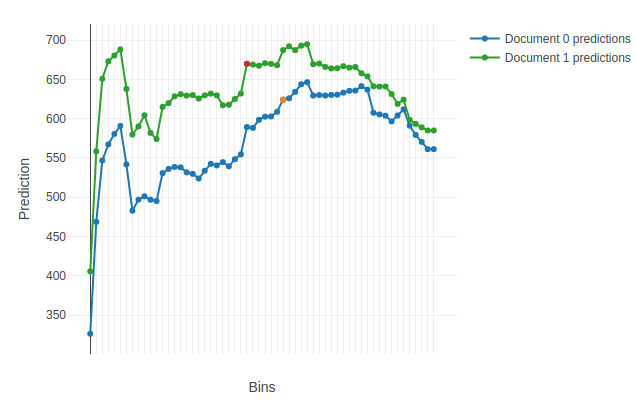plot_predictions

Sequentially vary the value of the specified features to put them into all buckets and calculate predictions for the input objects accordingly.

Restriction.
• Only models trained on datasets that do not contain categorical features are supported.
• Multiclassification modes are not supported.

Parameters

Parameter Possible types Description Default value
data
• numpy.array
• pandas.DataFrame
• catboost.Pool

The data to plot predictions for.

For example, use a two-document slice of the original dataset (refer to the example below).

Required parameter
features_to_change
• list of int
• string

• combination of list of int & string

The list of numerical features to vary the prediction value for.

For example, chose the required features by selecting top N most important features that impact the prediction results for a pair of objects according to PredictionDiff (refer to the example below).

Required parameter
plot bool

Plot a Jupyter Notebook chart based on the calculated predictions.

True
plot_file string

The name of the output HTML-file to save the chart to.

None (the files is not saved)
Parameter Possible types Description Default value
data
• numpy.array
• pandas.DataFrame
• catboost.Pool

The data to plot predictions for.

For example, use a two-document slice of the original dataset (refer to the example below).

Required parameter
features_to_change
• list of int
• string

• combination of list of int & string

The list of numerical features to vary the prediction value for.

For example, chose the required features by selecting top N most important features that impact the prediction results for a pair of objects according to PredictionDiff (refer to the example below).

Required parameter
plot bool

Plot a Jupyter Notebook chart based on the calculated predictions.

True
plot_file string

The name of the output HTML-file to save the chart to.

None (the files is not saved)

Type of return value

A list of dictionaries with predictions for all objects in the data float feature index -> [prediction for the object with corresponding feature values in the bucket : for all buckets used in the model]

Examples

import numpy as np
from catboost import Pool, CatBoost

train_data = np.random.randint(0, 100, size=(100, 10))
train_label = np.random.randint(0, 1000, size=(100))
train_pool = Pool(train_data, train_label)
train_pool_slice = train_pool.slice([2, 3])

model = CatBoost()
model.fit(train_pool)

prediction_diff = model.get_feature_importance(train_pool_slice,
type='PredictionDiff',
prettified=True)

model.plot_predictions(data=train_pool_slice,
features_to_change=prediction_diff["Feature Id"][:2],
plot=True,
plot_file="plot_predictions_file.html")

An example of the first plotted chart: